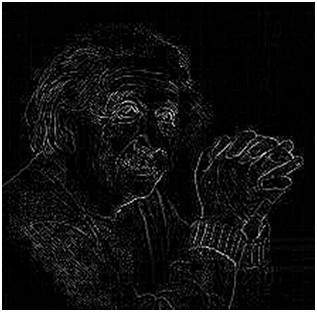# Laplacian Operator

Laplacian Operator is also a derivative operator which is used to find edges in an image. The major difference between Laplacian and other operators like Prewitt, Sobel, Robinson and Kirsch is that these all are first order derivative masks but Laplacian is a second order derivative mask. In this mask we have two further classifications one is Positive Laplacian Operator and other is Negative Laplacian Operator.

Another difference between Laplacian and other operators is that unlike other operators Laplacian didn’t take out edges in any particular direction but it take out edges in following classification.

• Inward Edges
• Outward Edges

Let’s see that how Laplacian operator works.

## Positive Laplacian Operator

In Positive Laplacian we have standard mask in which center element of the mask should be negative and corner elements of mask should be zero.

 0 1 0 1 -4 1 0 1 0

Positive Laplacian Operator is use to take out outward edges in an image.

## Negative Laplacian Operator

In negative Laplacian operator we also have a standard mask, in which center element should be positive. All the elements in the corner should be zero and rest of all the elements in the mask should be -1.

 0 -1 0 -1 4 -1 0 -1 0

Negative Laplacian operator is use to take out inward edges in an image

### How it works

Laplacian is a derivative operator; its uses highlight gray level discontinuities in an image and try to deemphasize regions with slowly varying gray levels. This operation in result produces such images which have grayish edge lines and other discontinuities on a dark background. This produces inward and outward edges in an image

The important thing is how to apply these filters onto image. Remember we can’t apply both the positive and negative Laplacian operator on the same image. we have to apply just one but the thing to remember is that if we apply positive Laplacian operator on the image then we subtract the resultant image from the original image to get the sharpened image. Similarly if we apply negative Laplacian operator then we have to add the resultant image onto original image to get the sharpened image.

Let’s apply these filters onto an image and see how it will get us inward and outward edges from an image. Suppose we have a following sample image.

### Sample Image### After applying Positive Laplacian Operator

After applying positive Laplacian operator we will get the following image.### After applying Negative Laplacian Operator

After applying negative Laplacian operator we will get the following image.